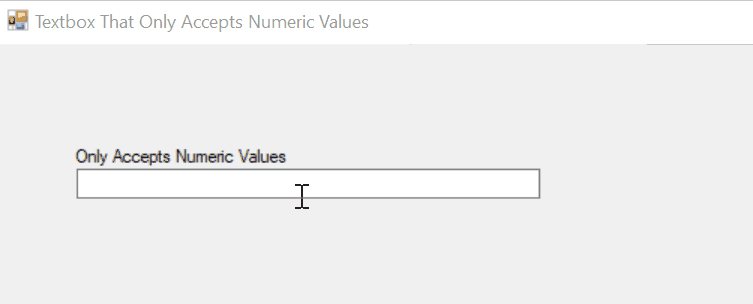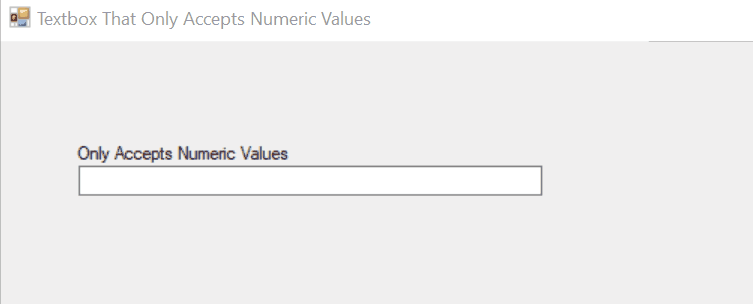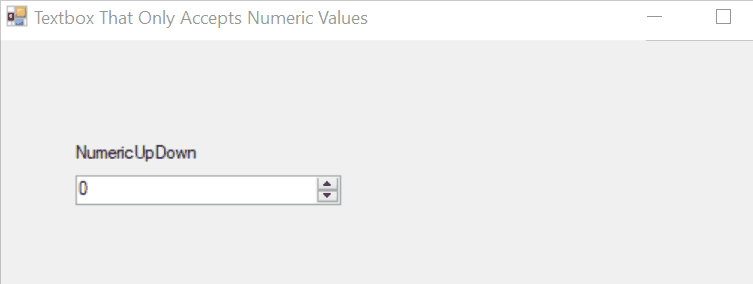# C# Make a Textbox That Only Accepts Numbers

Minahil Noor Oct 12, 2023

While making `Windows Forms`, some text fields only need a numeric value. For example, if we want to get the phone numbers from users then we will have to restrict our `textbox` to numeric values only.

In this article, we will focus on the methods that make a `textbox` that only accepts numbers.

## Make a `Textbox` That Only Accepts `Numbers` Using `KeyPressEventArgs` Class in `C#`

`KeyPressEventArgs` is a C# class that specifies the character entered when a user presses a key. Its `KeyChar` property returns the character that the user typed. Here we have used the `KeyPress` event to limit our `textbox` to numeric values only.

The key code that performs this action is as follows:

``````!char.IsControl(e.KeyChar) && !char.IsDigit(e.KeyChar) && (e.KeyChar != '.');
``````

Here `e` is a `KeyPressEventArgs` object that uses `KeyChar` property to fetch the entered key.

Example Code:

``````using System;
using System.Collections.Generic;
using System.ComponentModel;
using System.Data;
using System.Drawing;
using System.Linq;
using System.Text;
using System.Windows.Forms;
namespace WindowsFormsApplication1 {
public partial class Form1 : Form {
public Form1() {
InitializeComponent();
}
private void textBox1_KeyPress(object sender, KeyPressEventArgs e) {
if (!char.IsControl(e.KeyChar) && !char.IsDigit(e.KeyChar) && (e.KeyChar != '.')) {
e.Handled = true;
}

// only allow one decimal point
if ((e.KeyChar == '.') && ((sender as TextBox).Text.IndexOf('.') > -1)) {
e.Handled = true;
}
}
}
}
``````

Output:

``````//only allows numeric values in the textbox
``````## Make a `Textbox` That Only Accepts `Numbers` Using `Regex.IsMatch()` Method in `C#`

In C# we can use regular expressions to check various patterns. A regular expression is a specific pattern to perform a specific action. `RegularExpressions` is a C# class that contains the definition for `Regex.IsMatch()` method. In C#, we have `^[0-9]+\$` and `^\d+\$` regular expressions to check if a `string` is a number.

The correct syntax to use this method is as follows:

``````Regex.IsMatch(StringName, @"Expression");
``````

Example Code:

``````using System;
using System.Collections.Generic;
using System.ComponentModel;
using System.Data;
using System.Drawing;
using System.Linq;
using System.Text;
using System.Windows.Forms;
namespace WindowsFormsApplication1 {
public partial class Form1 : Form {
public Form1() {
InitializeComponent();
}
private void textBox1_TextChanged(object sender, EventArgs e) {
if (System.Text.RegularExpressions.Regex.IsMatch(textBox1.Text, "  ^ [0-9]")) {
textBox1.Text = "";
}
}
}
}
``````

Output:

``````//A textbox created that only accepts numbers.
``````## Make a `Textbox` That Only Accepts `Numbers` Using `NumericUpDown` Method

`NumericUpDown` provides the user with an interface to enter a numeric value using up and down buttons given with the `textbox`. You can simply drag and drop a `NumericUpDown` from the `Toolbox` to create a `textbox` that only accepts `numbers`.

You can also create a `NumericUpDown` object dynamically. The code to generate a `NumericUpDown` is as follows:

``````private System.Windows.Forms.NumericUpDown numericUpDown;
this.numericUpDown = new System.Windows.Forms.NumericUpDown();
``````

It has several properties which you can modify by opening the `Properties Windows`.

Output: